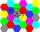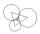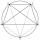# Rhombus construction

Construct ABCD rhombus if its diagonal AC=9 cm and side AB = 6 cm. Inscribe a circle in it touching all sides...

Result

x=##:  0

Leave us a comment of example and its solution (i.e. if it is still somewhat unclear...):

Showing 2 comments:Dr Math
1. draw line segment AB = 6 cm
2. draw circle k1 (B, r=6 cm)
2. draw circle k2 (A, r=9 cm)
4. point C is intersect k1 and k2
5. draw line u2 from B perpendicular to AC
6. draw circle k3 (A, r=6 cm)
7. point D is intersect k3 and line u2
8. connect points ABCD to rhombus
9. point S is intersect of AC diagonal and BD diagonal
10. make perpendicular line u3 from S to AB.
11. draw inswribed circle with center S and radius SX, X is intersection of u3 and ABDr Math
another steps:

1. Draw AC = 9cm horizontally.
2. With A as center and AB =6cm as radius, draw arcs above and below AC.
3. With C as center and AB as radius, draw arcs to cut the earlier arcs.
4. Intersection points are B and D.
5. Join all sides.
6. Draw BD. The intersection of AC AND BD is O , the center of the incircle.
7. Draw an arc with O as center and OB as radius to cut BC at E.
8. Draw perpendicular bisector of BE. It passes through O. Mid point of BE is F.
9. OF as radius and O as center draw the incircle.## Next similar examples:

1. The sum graphicallyDraw a graphically sum of the all sides of 4-gon ABCD.
2. Outer contact of circlesConstruct a circle k1 (S1; 1.5 cm), k2 (S2; 2 cm), and K3 (S3; 2.5 cm) so that they are always two outer contact. Calculate the perimeter of the triangle S1S2S3.
3. Regular octagonDraw the regular octagon ABCDEFGH inscribed with the circle k (S; r = 2.5 cm). Select point S' so that |SS'| = 4.5 cm. Draw S (S '): ABCDEFGH - A'B'C'D'E'F'G'H'.
4. 10 piecesHow to divide the circle into 10 parts (geometrically)?
5. Circular lawnAround a circular lawn area is 2 m wide sidewalk. The outer edge of the sidewalk is curb whose width is 2 m. Curbstone and the inner side of the sidewalk together form a concentric circles. Calculate the area of the circular lawn and the result round to 1
6. AceThe length of segment AB is 24 cm and the point M and N divided it into thirds. Calculate the circumference and area of this shape.
7. TreesAlong the road were planted 250 trees of two types. Cherry for 60 CZK apiece and apple 50 CZK apiece. The entire plantation cost 12,800 CZK. How many was cherries and apples?
8. Percentages52 is what percent of 93?
9. PersonsPersons surveyed:100 with result: Volleyball=15% Baseball=9% Sepak Takraw=8% Pingpong=8% Basketball=60% Find the average how many like Basketball and Volleyball. Please show your solution.
10. MushroomsEva and Jane collected 114 mushrooms together. Eve found twice as much as Jane. How many mushrooms found each of them?
11. EquationsSolve following system of equations: 6(x+7)+4(y-5)=12 2(x+y)-3(-2x+4y)=-44
12. Frameworks is badCalculate how many percent will increase the length of an HTML document, if any ASCII character unnecessarily encoded as hexadecimal HTML entity composed of six characters (ampersand, grid #, x, two hex digits and the semicolon). Ie. space as: &#x20;
13. Hotel roomsIn the 45 rooms, there were 169 guests, some rooms were three-bedrooms and some five-bedrooms. How many rooms were?
14. Two numbersWe have two numbers. Their sum is 140. One-fifth of the first number is equal to half the second number. Determine those unknown numbers.
15. TheatroTheatrical performance was attended by 480 spectators. Women were in the audience 40 more than men and children 60 less than half of adult spectators. How many men, women and children attended a theater performance?
16. The ballThe ball was discounted by 10 percent and then again by 30 percent. How many percent of the original price is now?
17. Three brothersThe three brothers have a total of 42 years. Jan is five years younger than Peter and Peter is 2 years younger than Michael. How many years has each of them?